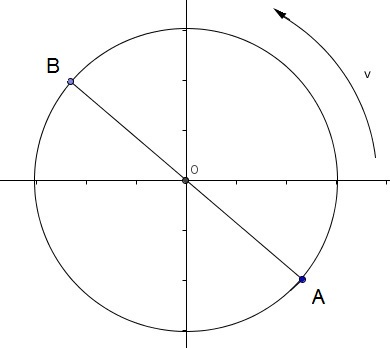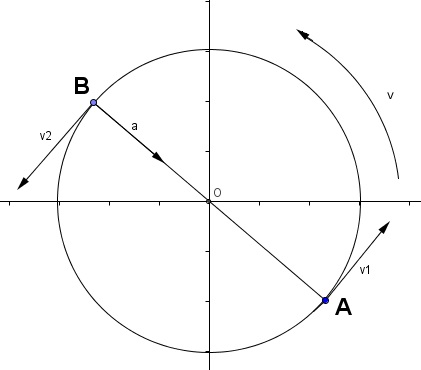# Solutions to Free SAT II Physics Practice Questions with Solutions Uniform Circular Motion

 Solutions to Sat Physics subject questions on uniform circular motion with detailed explanations. Fig. 1 below is related to questions 1, 2 and 3.Fig1. - Uniform Circular Motion. Fig.1 above refer to a point moving along a circular path. What is the direction of the velocity of the moving point at A?Solution - Explanations The direction is that of a tangent vector v1 at A pointing in the direction of motion as shown in the figure below. Answer DFig2. Fig.1 above refer to a point moving along a circular path. What is the direction of the centripetal acceleration at point B?Solution - Explanations The centripetal acceleration is vector a perpendicular to the (vector) velocity at point B and directed towards the center of the circle of rotation as shown in fig.2 above. Answer E. Fig.1 above refer to a point moving along a circular path. If BD is the diameter of the circle of rotation, then the velocities at A and B are A) equal in magnitude and have same direction B) equal in magnitude but have opposite direction C) not equal in magnitude but have the same direction D) not equal in magnitude but have opposite direction E) None of the above Solution - Explanations Again referring to fig.2 above, the velocities v1 at A and v2 at B have equal magnitude since the speed of the moving object is uniform and opposite direction since vectors v1 and v2 are both perpendicular to AB but directed towards the direction of rotation. Answer B. In a uniform circular motion, which of the following is constant? A) centripetal acceleration B) position C) velocity D) centripetal force E) speed Solution - Explanations By definition, in a uniform circular motion the speed is constant. Answer E. F is the centripetal force of a sphere rotating along a circular path at speed s. The mass of the sphere is m and is at distance R from the center. What is the centripetal force of the same sphere if the distance R is doubled? A) 2 F B) F / 2 C) F / 4 D) 4 F E) F / 3 Solution - Explanations Force F is given by F = m (s2 / R) Let us double R in the formula to obtain the new force F2 F2 = m (s2 / 2 R) = (1/2) m (s2 / R) = 1/2 F Answer B a is the centripetal acceleration of a sphere rotating along a circular path at speed s. The sphere is at distance R from the center. What is the centripetal acceleration of the same sphere if both distance R and speed s are doubled? A) a B) a / 4 C) a / 2 D) 2 a E) 4 a Solution - Explanations The formula for a is given by a = s2 / R Let us double R and s in the formula to obtain the new acceleration a2 a2 = (2 s)2 / (2 R) = 4 (s2) / (2 R) = 2 a Answer D What is the ratio s1 / s2 where s1 and s2 are the speeds of two satellites placed in stable circular orbits of radius R and 2R respectively? A) 2 B) √2 C) 1 / 2 D) 1 / √2 E) 2 √2 Solution - Explanations The centripetal force m s2 / R acting on the satellites is due to gravity G m M / R2. Hence m s2 / R = G m M / R2 where m is the mass of the satellite, s is the speed of the satellite, M the mass of the earth, R is the radius of the orbit and G is a constant. The above may be simplified to give s2 = G M / R For the two satellite we have: s12 = G M / R and s22 = G M / (2 R) Hence s1 / s2 = √( G M / R) / √( G M / 2 R ) = √ 2 Answer B What is the centripetal force acting on a car of mass 2000 Kg turning around a circular bend of radius 50 meters at the constant speed of 36 km/hr? A) 4,000 N B) 400 N C) 72,000 N D) 10,000 N E) 40 N Solution - Explanations For a moving object of mass m, at the constant speed s along a circle of radius R, the centripetal force is given by F = m s2 / R First convert speed from km/hr to m/s: 36 km / hour = 36 � 1000 m / 3600 s = 10 m/s F = (2000 Kg (10 m/s)2) / 50 m = 4,0000 Newtons Answer A What is the period of a point moving along a circular path of radius 5 cm at the linear speed of 200π cm / minutes? A) 10 s B) 300 s C) 180 s D) 40 se E) 3 s Solution - Explanations The period is the time taken for one whole rotation of the point around the circular path. The object rotates at the rate of 200π cm every minute or 60 seconds We need to find the time x for one rotation distance covered during one period (circumference) = 2π � 5 = 10π cm For 200π cm            time taken = 1 minute = 60 seconds For 10π cm            time taken = x = period Hence the period x = 60 s � 10π cm / 200π cm = 3 seconds Answer E A car is being driven on a road having two distant circular bends B1 and B2 of radius R and 3R respectively. If s1 is the speed of the car at the bend B1 and s2 is the speed at the bend B2, what should the ratio s1/s2 be so that the centripetal forces at both bends are equal? A) 1 B) √3 C) 1 / √3 D) 1 / √2 E) √2 Solution - Explanations Centripetal forces at F1 at B1 and F2 at B2 are given by F1 = m s12 / R , F1 = m s22 / (3R) , m is the mass of the car If F1 and F2 are equal, then m s12 / R = m s22 / (3R) Simplify and rewrite as s12 / s22 = R / (3R) = 1 / 3 s1 / s2 = 1 / √3 Answer C